## Assignments Fall 2011 (most recent at top)

#### [see Example] [see homework 2008] [fortran on the mac] [Return to main page]

 HW7 (final). Problem 1: (Hint: I think you can use spectralcalc for all of the calculated gas spectra and blackbody spectra). More hints: Discussion of the model used. Be sure to use spectralcalc to obtain atmospheric transmission, and then calculate the blackbody functions needed using the wavenumbers that come out of spectralcalc. Spectralcalc screens used for setting up the CO2 calculation are in the next two images. After your plot comes up, then save the text file as we have done in the past for other homework assignments. NOTE: Choose the 'triangle' instrument function with a width of 0.5 cm-1. That choice simulates what we can do, at best, with our FTIR spectrometer with regard to resolution, and it provides spectral smoothing of the high resolution calculation.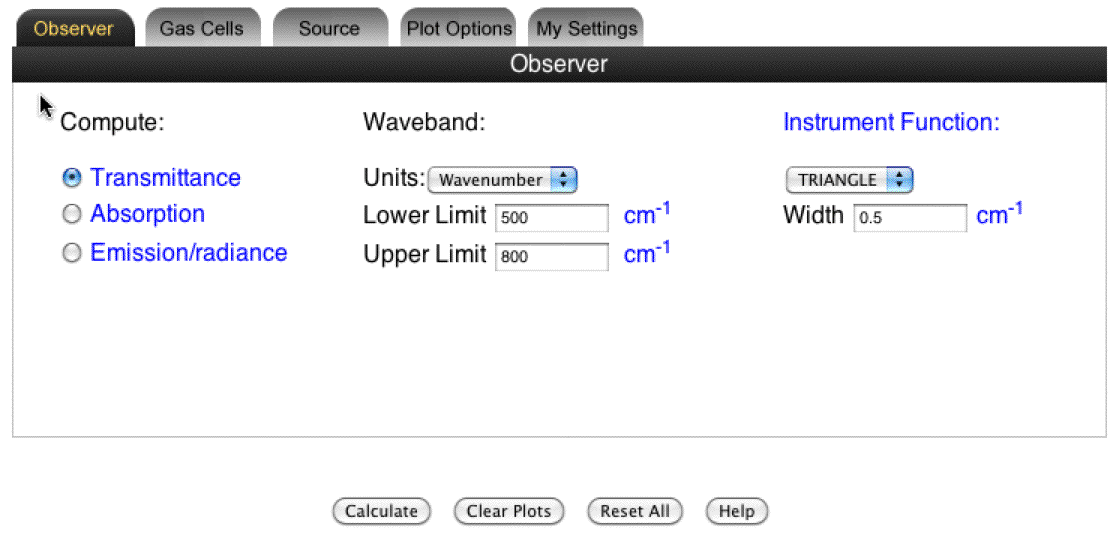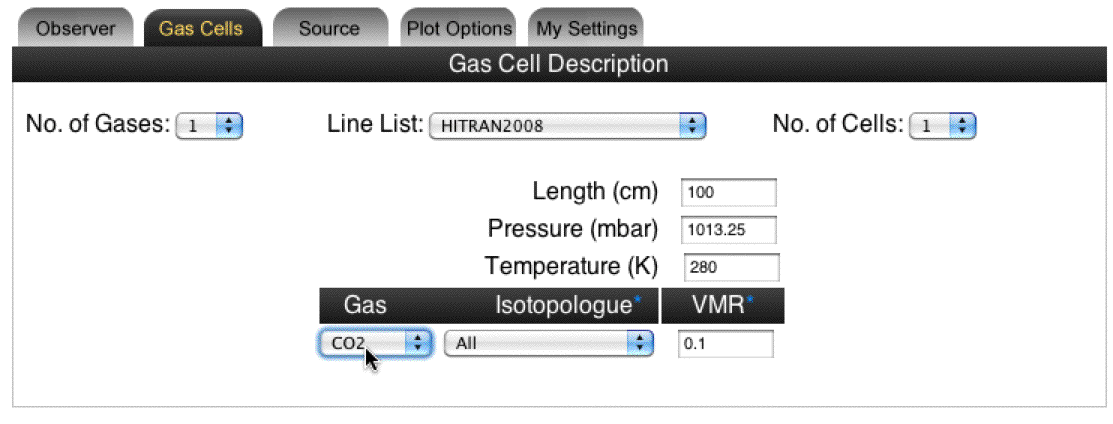Here is an example of what I got on the last part of the problem (CO2 and H20 together). Be sure to explain your results.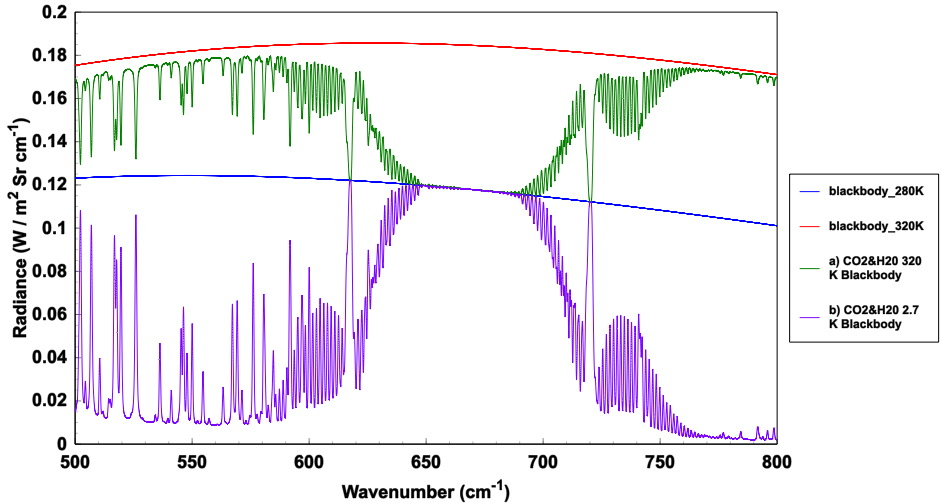OK: Here is the actual problem to solve: Calculate the spectral IR received at the surface and at a satellite for a simple model atmosphere. a) Reproduce figure 3 shown here, from this reference. Describe why the spectrum looks like it does. Is this a model for the surface IR or the satellite IR? (Here are my notes for IR calculation, and those from another software package.)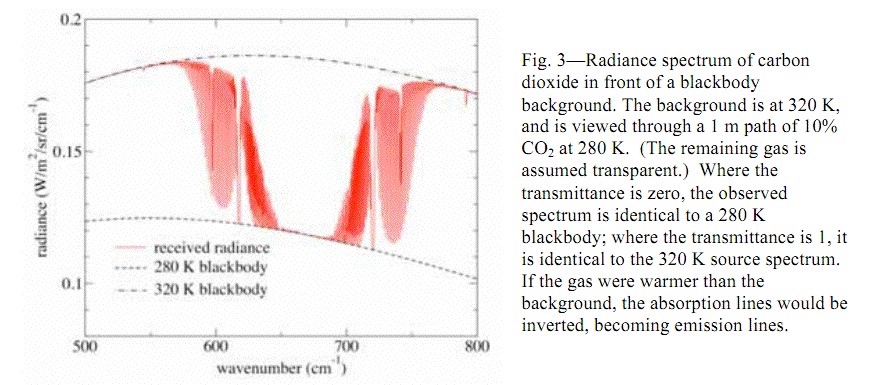b) Redo the calculation of a), but now using a background at 2.7 K instead of 320 K. Again, describe the spectrum, and answer the question, is this a model for the surface IR or the satellite IR? c) Redo the calculations of a) and b), only use water vapor only. d) Redo the calculations of a) and b), but use both water vapor AND CO2 in the calculation. Interpret the results in light of parts a) - c). PROBLEM 2. Aerosol radiative forcing (see this reference: note that the equation for backscatter is incorrect in this paper, but is correct below.)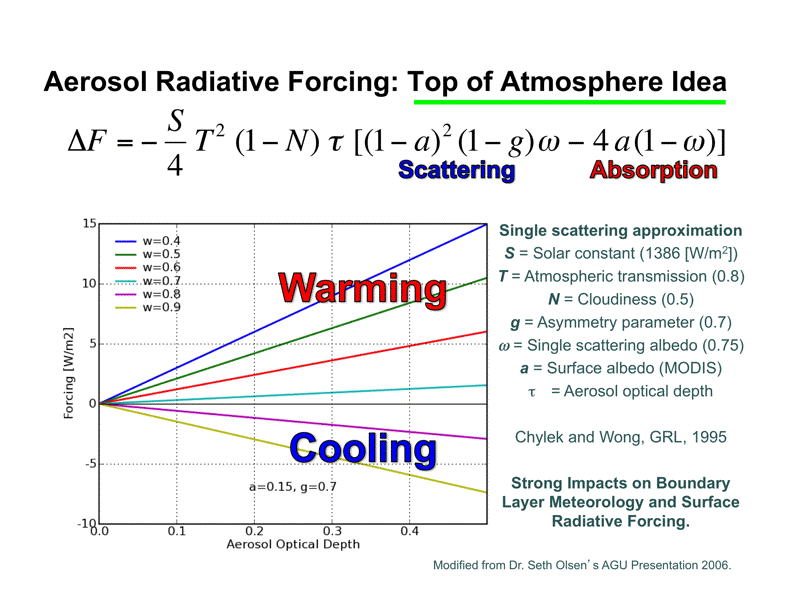Figure 1. Simple model for aerosol radiative forcing and an example. a) Show how the relationship given in Figure 1 above can be obtained from Eq. (8) of the reference.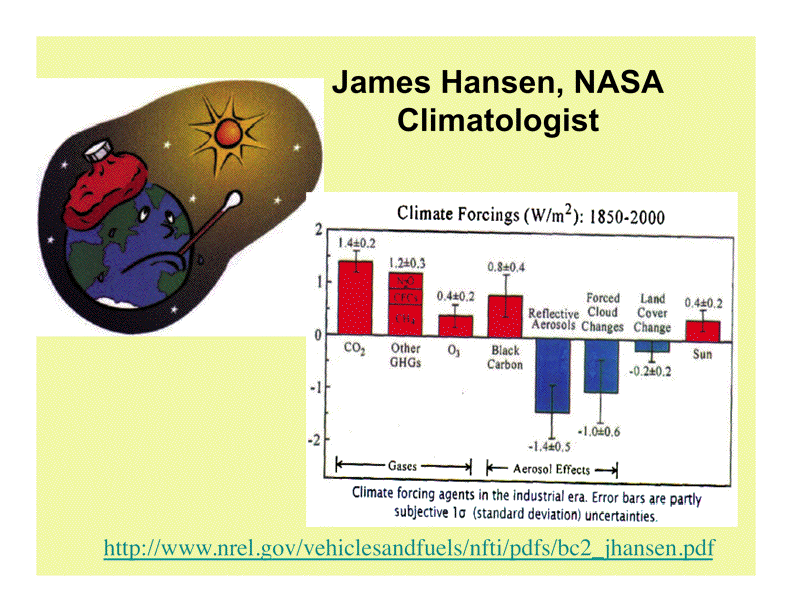Figure 2. Anthropogenic radiative forcing by aerosol. b) Assuming all of the same parameters for the model as given in Figure 1, except for aerosol optical depth and single scattering albedo, assume that all of the black carbon forcing in Figure 2 is due to the absorbing (warming) term in the model of figure 1, and that all of the 'reflective aerosols' forcing is due to the scattering (cooling) term. Determine an optical depth and single scattering albedo that explains these two aerosol radiative forcings. Are the values you determined likely to be observed in the atmosphere? c) Explain why top of the atmosphere radiative forcing described in Figures 1 and 2 don't really tell the whole story with respect to the importance of aerosol forcing on climate (hint: consider radiative forcing at the surface instead of the TOA, and think about planetary boundary layer dynamics.) d) Assuming an aerosol mass absorption efficiency of 8 square meters per gram, and a mass scattering efficiency of 4 square meters per gram, calculate the ratio of the absorbing (warming) term to the scattering (cooling) term as a function of the ratio of black carbon aerosol mass concentration divided by the total submicron aerosol mass concentration. Interpret. e) Bonus question: Derive Eq. (8) of the reference. Derive a similar expression for surface radiative forcing by aerosol. Problem 3) Refer to homework 4, part 2, problem 4 below. a) Warm up by redoing this part of the problem as stated here. Calculate the climate sensitivity to water droplet number by calculating dR/ dCCN. In words, how does the cloud albedo (reflectance) change with CCN? Assume all of the variation in R is due to CCN; hold all other parameters fixed. Explore and explain your solution as a function of total optical depth τ. b) Repeat the calculation, only this time for dR/dLWP and then dR/dRe, where LWP is liquid water path, and Re is effective radius. Interpret your results. Of CCN, LWP, and Re, which term most strongly affects cloud albedo R? Interpret. Problem 4) What are the P, Q, and R branches of the absorption spectra by CO2? Problem 5) Consider these two articles (and any other information you can find on these subjects). a) What is collision induced absorption? Is it a significant factor in the Earth's radiative balance? Is it useful for remote sensing applications? b) After appreciating the vertical distribution of dust and ozone in the atmosphere, is it likely that ozone concentration is affected by dust concentration? (This article, for those interested, gives details of the balloon sounder.)

 HW6. Description is given here. Infrared radiation in the atmosphere, and calculation of atmospheric heating rate when an absorber (black carbon) is constant. HELP ON THE TRANSMISSION CALCULATION, LAST PROBLEM : Concise theory. Another description of the theory, less detailed, but more general. On the CO2 problem(s), you can use spectralcalc to retrieve the line parameters. Here are the screens that will help you set up spectralcalc for the calculation.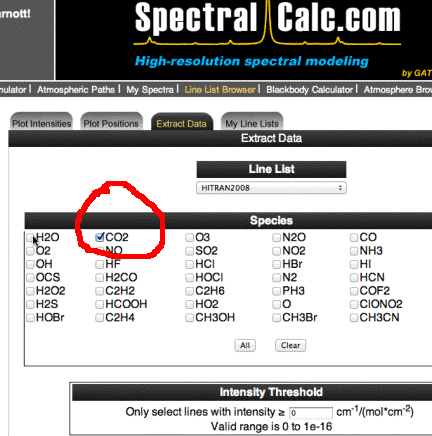SCREEN 1.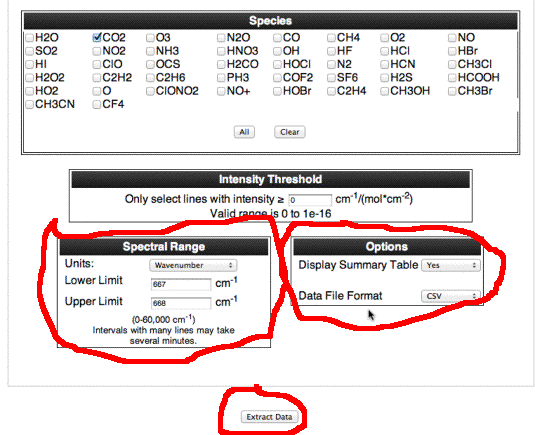SCREEN 2. NOTE: Choose the spectral range specific for your problem.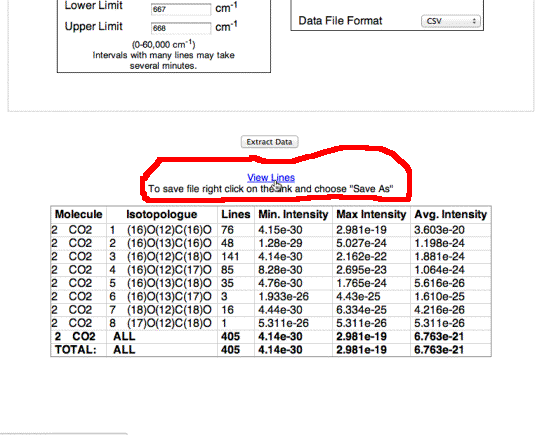SCREEN 3. Once you are done with screen 3, then you should have a comma separated list of line parameters. Copy and paste them to your text editing program (notepad on the pc, I use textwrangler for the mac). Save this file and then read it into Excel or other program so that it will nicely put the file in order so that you can extract the line strength and the air broadened half width (as well as the wavenumber). Look for the specific wavenumber referred to in the problem. May also find this general problem of flux and cross section calculation to be useful.

 HW5. Atmospheric Optics, rainbow and glory. See notes page for week 10 for description. Gronstal solution.

 HW4. USE the multiple scattering paper by Bohren and find a Mie theory calculator (code) for scattering and absorption by spheres. Also read the cloud/aerosol interaction climate paper by Han and Rossow and others. Supplemental reading: Chapter 13 of Petty; Chapter 7 of Stamnes and Thomas. HOMEWORK 4: Part 1. HOMEWORK 4: Part 2. They illustrate many features of the cloud aerosol interaction and cloud albedo. Solution for the Twomey effect calculation problem.

 HW3. Read the multiple scattering paper by Bohren. Supplemental reading: Chapter 13 of Petty; Chapter 7 of Stamnes and Thomas. Do these 4 problems. They illustrate many features of multiple scattering and cloud albedo.

HW2. Read chapter 2. You may also find it helpful to read Chapter 2 of Petty as well (highly recommended!)

Consider these questions for review and class discussion.

In your own words, express what is meant by flux, net flux, irradiance, radiance, and describe circumstances when we would want to use each.

Problems to turn in. Do problem 3 first.

Extra Credit except for Ian and Nic: Ian and Nic will demonstrate its solution to us (it is related to their research with nephelometer development). : Problem 1. Do problem 2.3.

Problem 2. This problem is designed to give you experience calculating cloud optical depths in the UV, visible, and near IR wavelength ranges, and to introduce you to the single scattering albedo.

Consider a cumulus cloud composed of cloud droplets all having diameters of D=20 microns. Let there be N cloud droplets per volume, and specifically, N=200 / cc for our cloud. Single scattering albedo, w, is defined as the scattering coefficient divided by the extinction coefficient. The extinction coefficient is the sum of the scattering and absorption coefficients. Furthermore, the extinction cross section per droplet can be simply taken to be twice the droplet projected area for UV, visible, and near IR wavelengths. See class notes where this was discussed.

a. Derive general expressions for the mean free path for extinction, scattering, and absorption events, in terms of the extinction coefficient and single scattering albedo. By definition the optical depth for the cloud reaches unity (i.e. 1) when radiation has penetrated into the cloud a distance equal to the mean free path. We often call this distance the electromagnetic skin depth as well.

b. Suppose that we looked at the cloud as a continuous medium. Obtain a relationship for the imaginary part of the refractive index at wavelength lambda for this 'equivalent' medium such that the absorption optical depth is the same as in part a) for a distance equal to the penetration depth. We will discuss the refractive index for continuous media using this presentation.

c. Calculate actual values for all derived parameters in parts a and b for single scattering albedo equal to 1, 0.999, 0.8, and 0. Interpret especially the extreme cases.

d.What does the skin depth calculation have to do with satellite remote sensing and ground based remote sensing of clouds? What role does multiple scattering play here for this question? (hint: scattering optical depths greater than unity require use of multiple scattering theory for calculating transmission and reflection of light by clouds.)

Problem 3. Real world optical depth calculation for column ozone, water vapor, oxygen, and nitrogen dioxide. (This is a very timely subject for Marce as her research is centered on use of sun photometers for remote sensing of atmospheric properties.)

(For reference, measured gaseous absorption cross sections are available from here.)

We will attempt to understand atmospheric optical depth measurements made here in Reno with our spectrometer based sun photometer. Here is a graphic of those measurements. We will be working only with the AOD curve, ignore the others for now.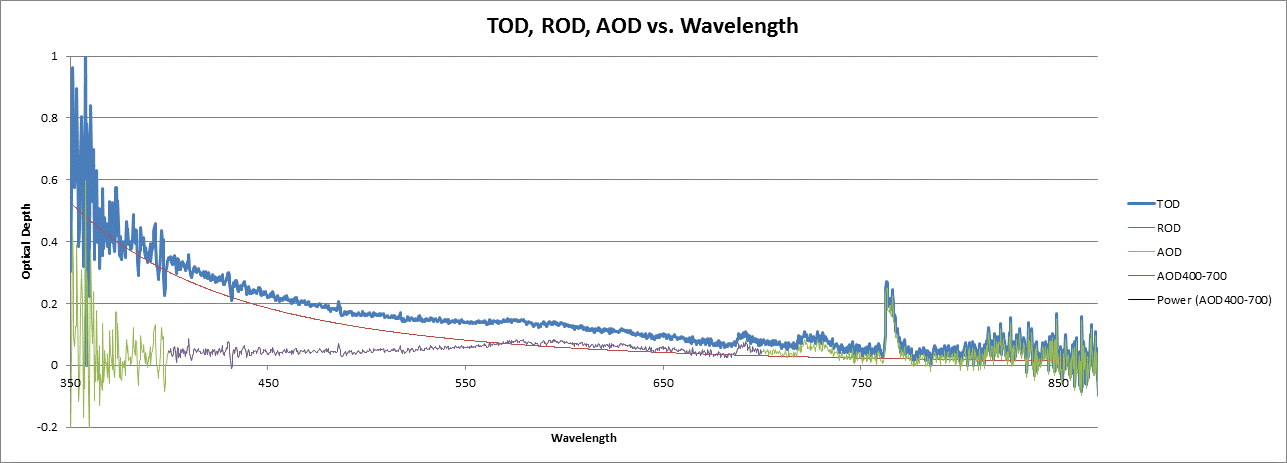The experimentally measured sun photometer spectral data is here in excel format, and in text format. We will be working with the curve and column of data labeled "AOD", where AOD refers to aerosol optical depth. The fundamental problem is that the AOD measurements are in fact actually also affected by absorption of sunlight by gases in the atmosphere, especially ozone (Chappius band), water vapor, molecular oxygen, and nitrogen dioxide. Our goals here are to become familiar with measured spectra (with all of its quirks), and to simulate the gaseous absorption part of the AOD curves.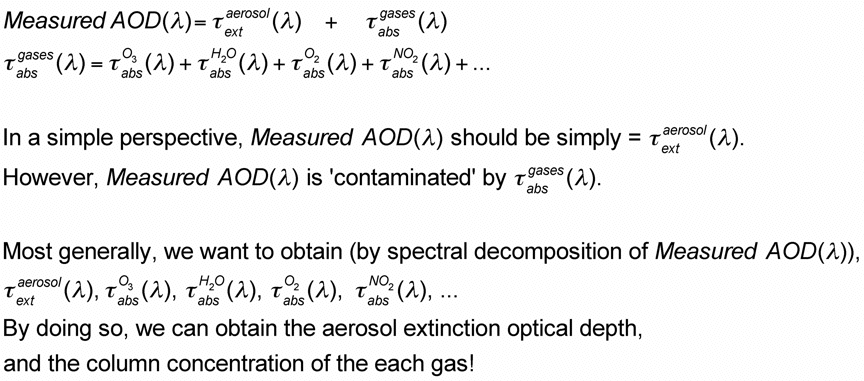Here is a discussion on how to calculate absorption optical depth for various gases.

Parts (a) through (e) are simply about plotting the cross section spectra from each gas,
and some explanation of where they came from is in each step.

If you are going to do part (h) as extra credit, then you should obtain the cross sections for each gas from the links given in parts (a) through (d). If you prefer not to do the extra credit problem, then you can just use the averaged cross section spectra collected in this file.

(a) Use the ozone cross section per molecule; data for 280 K and 1000 mb (this data was from here: data by Voigt).
Make a plot of the absorption cross section per molecule for the wavelength range 350 nm to 850 nm. What is the name of this band of ozone absorption? At what wavelength does it peak? Make a linear plot (rather than logarithmic).

(b) Use the oxygen cross section per molecule for 293 K and the near IR (this data was obtained here: data by Bogumil). Add this data to your plot from part (a). You may have to multiply the cross section column for O2 by a factor of 1000 or so to make it a reasonably comparable with the O3 cross section.

(c) Use the NO2 cross section per molecule for visible wavelengths (this data was obtained from here: presumably 293 K) (data by Vandaele). Add this data to your plot from part (a). You may have to divide the data by 200 to make the plot work with the O3 cross section.

(d) Find the H20 cross section per molecule for visible and near IR wavelengths (calculated using spectralcalc).
Add this data to your plot from part (a). Here is a movie describing how I did the calculations using spectralcalc, and using this fortran code.

(e) Now look at your overall plot. It should look more or less something like this (I plotted the raw spectra here, so yours will likely be smoother).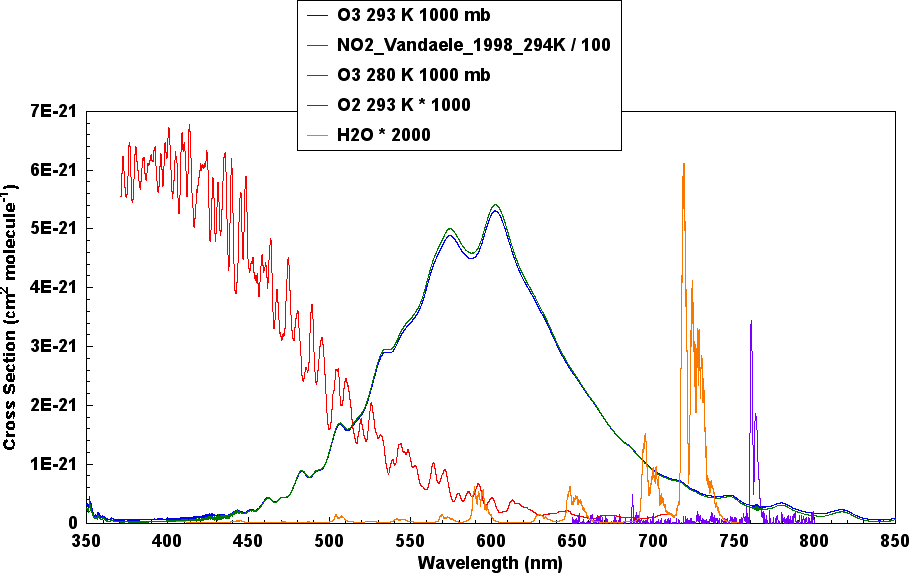(f) Now calculate optical depths for each gas as a function of wavelength. (Theory is given here).
Then express the water vapor column optical depth in terms of (mm) of precipitable water (perhaps 10 mm).
Then express the O3 column concentration in Dobson units (perhaps 300).
Use 21% oxygen, and an NO2 concentration of 0.1 ppb = 1x10-9.
Make an overlay graph of the gaseous optical depth spectra, and the sum of all of them.
In what spectral ranges do the various gases dominate absorption?

(g) Manually adjust the concentration of each gas to see if you can explain various features of the gaseous absorption part of the measured AOD spectrum. What are the gas concentrations you come up with? Are they reasonable?

Here is an example from what I obtained. Oxygen was too high by a factor of about 35 so I adjusted it down by that factor. NO2 doesn't really matter that much so it's hard to assess its accuracy. Anyone that can resolve the oxygen issue gets a special prize..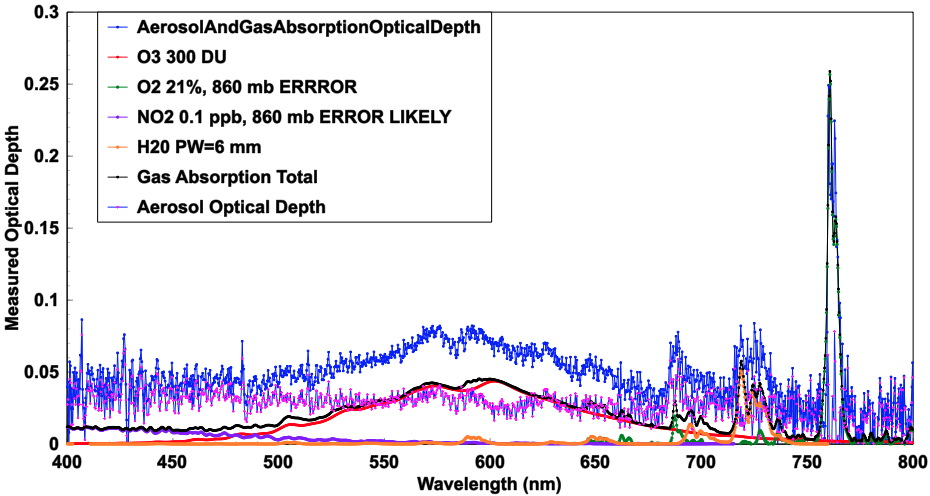### EXTRA CREDIT: AND HIGHLY RECOMMENDED FOR THOSE DOING SUN PHOTOMETER RESEARCH.

(h) The high resolution gaseous laboratory cross section spectra are here. In general the total optical depth should be obtained first, and then the spectral averaging done second. As an approximation we can just bring the cross section spectra of problem (e) to the same resolution as the observed optical depth spectrum from our spectrometer based sun photometer. Then calculate the optical depths again as in part (f) and add them together (as an approximation) to get the total gaseous absorption optical depth. Theory for spectral averaging can be found as part of this document, or more simply just the relevant portion of the document here. (Note: here is the result of doing this calculation. The program used for doing the spectral average is here.).

 Fortran on the mac: An example fortran subroutine for implementing spectal averaging is here. Fortran can be installed for the mac as described here. Be sure to also install XCODE application for the mac using the App Finder. (One note to solve problems). It is very convenient to use TextWrangler on the mac for editing filename.f fortran programs. Here are my .login and brief compile script I use for creating executable programs from fortran source code.